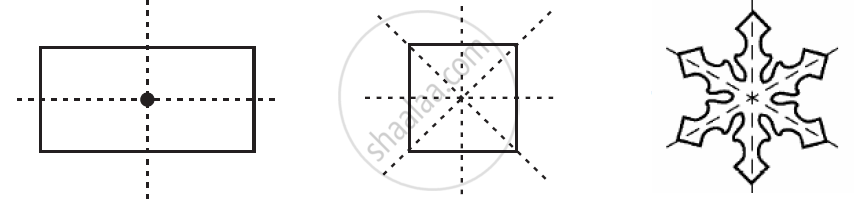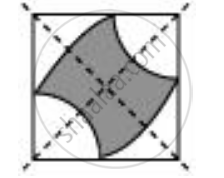Share

# The Following Figures Have More than One Line of Symmetry. Such Figures Are Said to Have Multiple Lines of Symmetry Identify Multiple Lines of Symmetry, If Any, in the Given Figure-4 - CBSE Class 7 - Mathematics

ConceptLines of Symmetry for Regular Polygons

#### Question

The following figures have more than one line of symmetry. Such figures are said to have multiple lines of symmetryIdentify multiple lines of symmetry, if any, in the given figure#### Solution

The given figure has 2 lines of symmetry. Hence, it has multiple lines of symmetryIs there an error in this question or solution?

#### APPEARS IN

NCERT Solution for Mathematics for Class 7 (2018 to Current)
Chapter 14: Symmetry
Ex. 14.10 | Q: 4.4 | Page no. 269
Solution The Following Figures Have More than One Line of Symmetry. Such Figures Are Said to Have Multiple Lines of Symmetry Identify Multiple Lines of Symmetry, If Any, in the Given Figure-4 Concept: Lines of Symmetry for Regular Polygons.
S